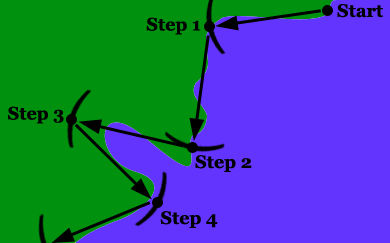HandsOn 1 - "Walking" Along a CoastlineFigure 2.1: Measuring a coastline with caliper. The caliper often "steps across'' a peninsula or inlet.

Table 2.1: Measuring the length of a coastline.

 1. Size of step, S 2. PREDICTED 3. MEASURED 4. "Length'' = N×S Number of Steps, N Number of Steps, N ×××××

To investigate coastlines, start by "walking'' along the map of a coastline as shown in Figure 2.1.

You will need:

• a map of the coastline to be measured and
• a pair of calipers, i.e., dividers (a caliper or divider is like a circle-drawing compass, except that both ends have points)

A. the points on the map between which to measure the length of coastline
B. the scaled distance on the map for the first setting between the ends of the calipers (preferably a multiple of two, such as 64 kilometers or 32 miles).

2. Separate the points of the calipers by the scaled distance of step 1B. Place the first end of the calipers at the beginning of the coastline to be measured and swing your calipers so that the second end rests on the coastline as close to the starting point as possible. This is your first step along the coastline.

3. Now swing the caliper so that the first end "walks'' along to the nearest next point on the coastline. This is your second step.

4. Continue this process, counting the steps as you go; stop when your next step would carry you beyond the end of the coastline. The total number of steps multiplied by the distance covered in each step gives an initial measurement of the length of your coastline. For example: 10 steps, each of a length of 64 kilometers, yields a total length of 10×64 kilometers = 640 kilometers.

5. Enter the size of the step (in miles, kilometers, or whatever) in the left column of your copy of Table 2.1. Enter the number of steps in the third column.

6. Now you are going to start again, this time reducing the distance between the calipers to half its original value. Before you do this, predict the number of steps it will take you to walk along the coastline with this smaller step size. Enter your prediction in the second column of your copy of the table.

7. "Walk'' along the coastline again with the calipers set to half the original distance. Count the total number of steps and record the results in the correct columns of your copy of Table 2.1. How accurate was your predicted number of steps?

8. Repeat the process of cutting in half the distance between the caliper points as many times as you can, each time making a prediction, taking the walk, and filling in your copy of the table with your results.

9. Fill in the right-hand column of your copy of the table by multiplying each step size by the number of steps to get a total "length.''

 Q2.2: Did your prediction of the number of steps become more accurate with each new setting of the calipers?

 Q2.3: What happens to the total length of the coastline for different step sizes?

 Q2.4: What would you estimate the "final length'' of the coastline would converge to as the step size gets smaller and smaller?

Previous: 2.1 - Coastline

Next: HandsOn 2 - Measuring the Dimension of a Coastline